### Life Mathematics Class 8th Mathematics Term 3 Tamilnadu Board Solution

##### Question 1.A man can complete a work in 4 days, whereas a woman can complete it in only12 days. If they work together, in how many days, can the work be completed?Answer:A man can complete a work in 4 daysMan’s 1-day work =Woman can complete a work in 12 daysWoman’s 1-day work =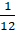1-day work of both =+=By working together, they can complete a work in 3 daysQuestion 2.Two boys can finish a work in 10 days when they work together. The first boy can do it alone in 15 days. Find in how many days will the second boy do it all himself?Answer:If two boys work together they can finish a work in 10 daysLet the two boys be x & yx & y’s 1-day work =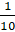x + y =..eq (1)first boy (x) can do it in 15 daysx’s 1-day work =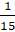x =substituting the value of x in eq(1)x + y =+ y =y =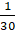y’s 1-day work =so, second boy (y) can do a work in 30 daysQuestion 3.Three men A, B and C can complete a job in 8, 12 and 16 days respectively.A and B work together for 3 days; then B leaves and C joins. In how many days, can A and C finish the work?Answer:A, B and C can complete a job in 8, 12 and 16 days respectively.1-Day work:A’s =B’s =C’s =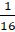A and B’s 1-day work =+=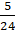A and B work together for 3 days= 3 ×=The remaining work after 3 days = 1-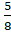=The remaining work will be done by A & C togetherWork done by A & C =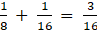By doing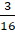potion of the work in a day they can complete remaining work (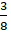) in 2 daysQuestion 4.A tap A can fill a drum in 10 minutes. A second tap B can fill in 20 minutes. A third tap C can empty in 15 minutes. If initially the drum is empty, find when it will be full if all taps are opened together?Answer:Time taken by A = 10 minWork done by A in 1 min =Time taken by B = 20 minWork done by B in 1 min =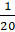Time taken by C = 15 minWork done by C in 1 min =Work done by all three A, B & C in 1 min =+-=Time taken by all three A, B & C in = 12 minQuestion 5.A can finish a job in 20 days and B can complete it in 30 days. They work together and finish the job. If Rs. 600 is paid as wages, find the share of each.Answer:Time taken by A = 20 daysWork done by A in 1 day =Time taken by B = 30 daysWork done by B in 1 day =Wages = 600Ratio of work done by A & B in 1-day =:= 3:2So. The ratio of the wages will be 3:23x + 2x = 6005x = 600X = 120A’s wage = 3x = 3 × 120 = 360B’s wage = 2x = 2 × 120 = 240Question 6.A, B and C can do a work in 12, 24 and 8 days respectively. They all work for one day. Then C leaves the group. In how many days will A and B complete the rest of the work?Answer:A, B and C can do a work in 12, 24 and 8 days respectively.A, B and C’s 1-day work will be,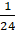,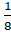respectively1-day’s work of all of them =++=Remaining portion of work = 1-=After that C leaves the group. So, the rest of the work will be completed by A & B onlyA & B’s 1-day’s work =+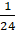=No. of day required =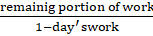=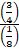= 6 daysQuestion 7.A tap can fill a tank in 15 minutes. Another tap can empty it in 20 minutes. Initially the tank is empty. If both the taps start functioning, when will the tank become full?Answer:Time taken by A = 15 minWork done by A in 1 min =Time taken by B = 30 minWork done by B in 1 min =Work done by all three A, B in 1 min =-=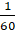(∵ B empties the tank we use negative there)Time taken to fill to the tank is 60 min

PDF FILE TO YOUR EMAIL IMMEDIATELY PURCHASE NOTES & PAPER SOLUTION. @ Rs. 50/- each (GST extra)

HINDI ENTIRE PAPER SOLUTION

MARATHI PAPER SOLUTION

SSC MATHS I PAPER SOLUTION

SSC MATHS II PAPER SOLUTION

SSC SCIENCE I PAPER SOLUTION

SSC SCIENCE II PAPER SOLUTION

SSC ENGLISH PAPER SOLUTION

SSC & HSC ENGLISH WRITING SKILL

HSC ACCOUNTS NOTES

HSC OCM NOTES

HSC ECONOMICS NOTES

HSC SECRETARIAL PRACTICE NOTES

# 2019 Board Paper Solution

HSC ENGLISH SET A 2019 21st February, 2019

HSC ENGLISH SET B 2019 21st February, 2019

HSC ENGLISH SET C 2019 21st February, 2019

HSC ENGLISH SET D 2019 21st February, 2019

SECRETARIAL PRACTICE (S.P) 2019 25th February, 2019

HSC XII PHYSICS 2019 25th February, 2019

CHEMISTRY XII HSC SOLUTION 27th, February, 2019

OCM PAPER SOLUTION 2019 27th, February, 2019

HSC MATHS PAPER SOLUTION COMMERCE, 2nd March, 2019

HSC MATHS PAPER SOLUTION SCIENCE 2nd, March, 2019

SSC ENGLISH STD 10 5TH MARCH, 2019.

HSC XII ACCOUNTS 2019 6th March, 2019

HSC XII BIOLOGY 2019 6TH March, 2019

HSC XII ECONOMICS 9Th March 2019

SSC Maths I March 2019 Solution 10th Standard11th, March, 2019

SSC MATHS II MARCH 2019 SOLUTION 10TH STD.13th March, 2019

SSC SCIENCE I MARCH 2019 SOLUTION 10TH STD. 15th March, 2019.

SSC SCIENCE II MARCH 2019 SOLUTION 10TH STD. 18th March, 2019.

SSC SOCIAL SCIENCE I MARCH 2019 SOLUTION20th March, 2019

SSC SOCIAL SCIENCE II MARCH 2019 SOLUTION, 22nd March, 2019

XII CBSE - BOARD - MARCH - 2019 ENGLISH - QP + SOLUTIONS, 2nd March, 2019

# HSCMaharashtraBoardPapers2020

(Std 12th English Medium)

HSC ECONOMICS MARCH 2020

HSC OCM MARCH 2020

HSC ACCOUNTS MARCH 2020

HSC S.P. MARCH 2020

HSC ENGLISH MARCH 2020

HSC HINDI MARCH 2020

HSC MARATHI MARCH 2020

HSC MATHS MARCH 2020

# SSCMaharashtraBoardPapers2020

(Std 10th English Medium)

English MARCH 2020

HindI MARCH 2020

Hindi (Composite) MARCH 2020

Marathi MARCH 2020

Mathematics (Paper 1) MARCH 2020

Mathematics (Paper 2) MARCH 2020

Sanskrit MARCH 2020

Sanskrit (Composite) MARCH 2020

Science (Paper 1) MARCH 2020

Science (Paper 2)

Geography Model Set 1 2020-2021

MUST REMEMBER THINGS on the day of Exam

Are you prepared? for English Grammar in Board Exam.

Paper Presentation In Board Exam

How to Score Good Marks in SSC Board Exams

Tips To Score More Than 90% Marks In 12th Board Exam

How to write English exams?

How to prepare for board exam when less time is left

How to memorise what you learn for board exam

No. 1 Simple Hack, you can try out, in preparing for Board Exam

How to Study for CBSE Class 10 Board Exams Subject Wise Tips?

JEE Main 2020 Registration Process – Exam Pattern & Important Dates

NEET UG 2020 Registration Process Exam Pattern & Important Dates

How can One Prepare for two Competitive Exams at the same time?

8 Proven Tips to Handle Anxiety before Exams!